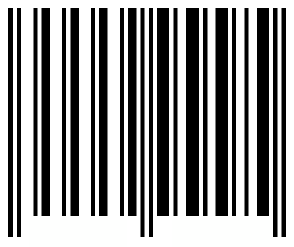2.25

Weizmann Institute of Science

Skip to 0 minutes and 0 secondsWe have got to the time of the course where we meet our alien challenge again. Well, we've nearly finished the alien challenge. We only have the last row. This is what we've learned so far. I times KINGJO equals JOKING, where I = 4 We deciphered this to mean 4 times 142857 = 571,428 and this is a cyclic number if you recall. We also know that 7 in a house is equal to 142, 857 which is equal to 7 in a house - this is just the Gray code for 7. and that's equal to - in Gray code - this number is equal to 999,999 divided by 7. And now we have the last row.

Skip to 0 minutes and 54 seconds7 in a house times 7 equals a barcode. Can you decipher the barcode?

Barcode alien challenge